GeeksforGeeks App
Open AppBrowser
Continue

# Code Converters – Binary to/from Gray Code

Prerequisite – Number System and base conversions

Gray Code system is a binary number system in which every successive pair of numbers differs in only one bit. It is used in applications in which the normal sequence of binary numbers generated by the hardware may produce an error or ambiguity during the transition from one number to the next.
For example, the states of a system may change from 3(011) to 4(100) as- 011 — 001 — 101 — 100. Therefore there is a high chance of a wrong state being read while the system changes from the initial state to the final state.
This could have serious consequences for the machine using the information. The Gray code eliminates this problem since only one bit changes its value during any transition between two numbers.

### Converting Binary to Gray Code –

Letbe the bits representing the binary numbers, whereis the LSB andis the MSB, and
Letbe the bits representing the gray code of the binary numbers, whereis the LSB andis the MSB.
The truth table for the conversion is-To find the corresponding digital circuit, we will use the K-Map technique for each of the gray code bits as output with all of the binary bits as input.
K-map for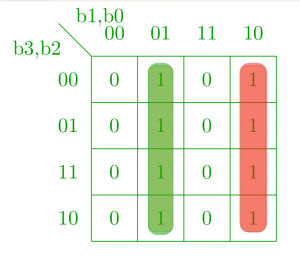K-map for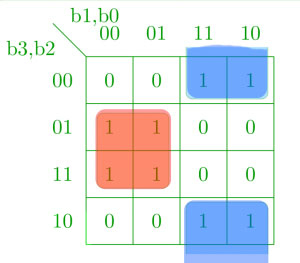K-map for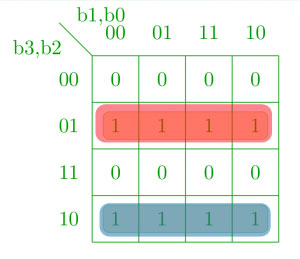K-map for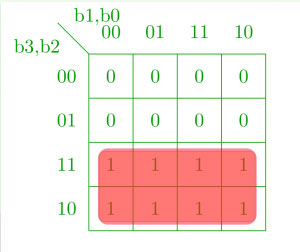Corresponding minimized boolean expressions for gray code bits –The corresponding digital circuit –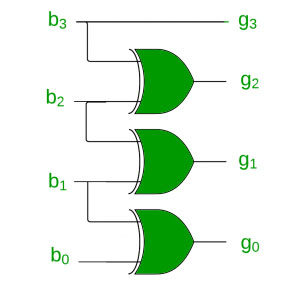### Generalized Boolean Expression for conversion of Binary to Gray Code –

Boolean expression for conversion of binary to gray code for n-bit :

G n = B n

G n-1 = B n XOR B n-1 : :

G 1 = B 2 XOR B 1

### Converting Gray Code to Binary –

Converting gray code back to binary can be done in a similar manner.
Letbe the bits representing the binary numbers, whereis the LSB andis the MSB, and
Letbe the bits representing the gray code of the binary numbers, whereis the LSB andis the MSB.
Truth table-Using K-map to get back the binary bits from the gray code –
K-map for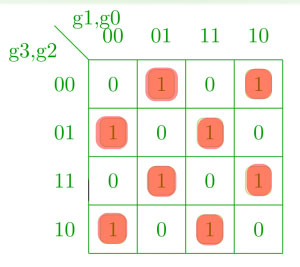K-map for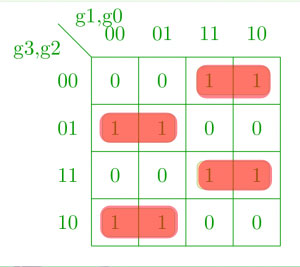K-map for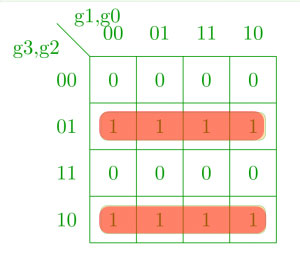K-map for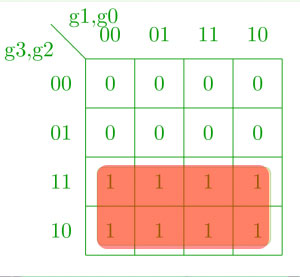Corresponding Boolean expressions –Corresponding digital circuit –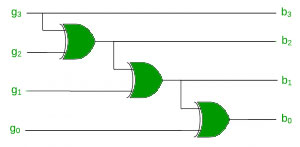### Generalized Boolean Expression for conversion of Gray to Binary Code –

Boolean expression for conversion of gray to binary code for n-bit :

B n = G n

B n-1 = B n XOR G n-1 = G n XOR G n-1 : :

B 1 = B 2 XOR G 1 = G n XOR ………… XOR G 1

This article is contributed by Chirag Manwani. If you like GeeksforGeeks and would like to contribute, you can also write an article using write.geeksforgeeks.org or mail your article to review-team@geeksforgeeks.org. See your article appearing on the GeeksforGeeks main page and help other Geeks.